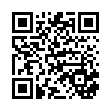# final (PDF)

### File information

This PDF 1.5 document has been generated by TeX / MiKTeX pdfTeX-1.40.14, and has been sent on pdf-archive.com on 19/05/2016 at 17:38, from IP address 24.149.x.x. The current document download page has been viewed 594 times.
File size: 148.89 KB (14 pages).
Privacy: public file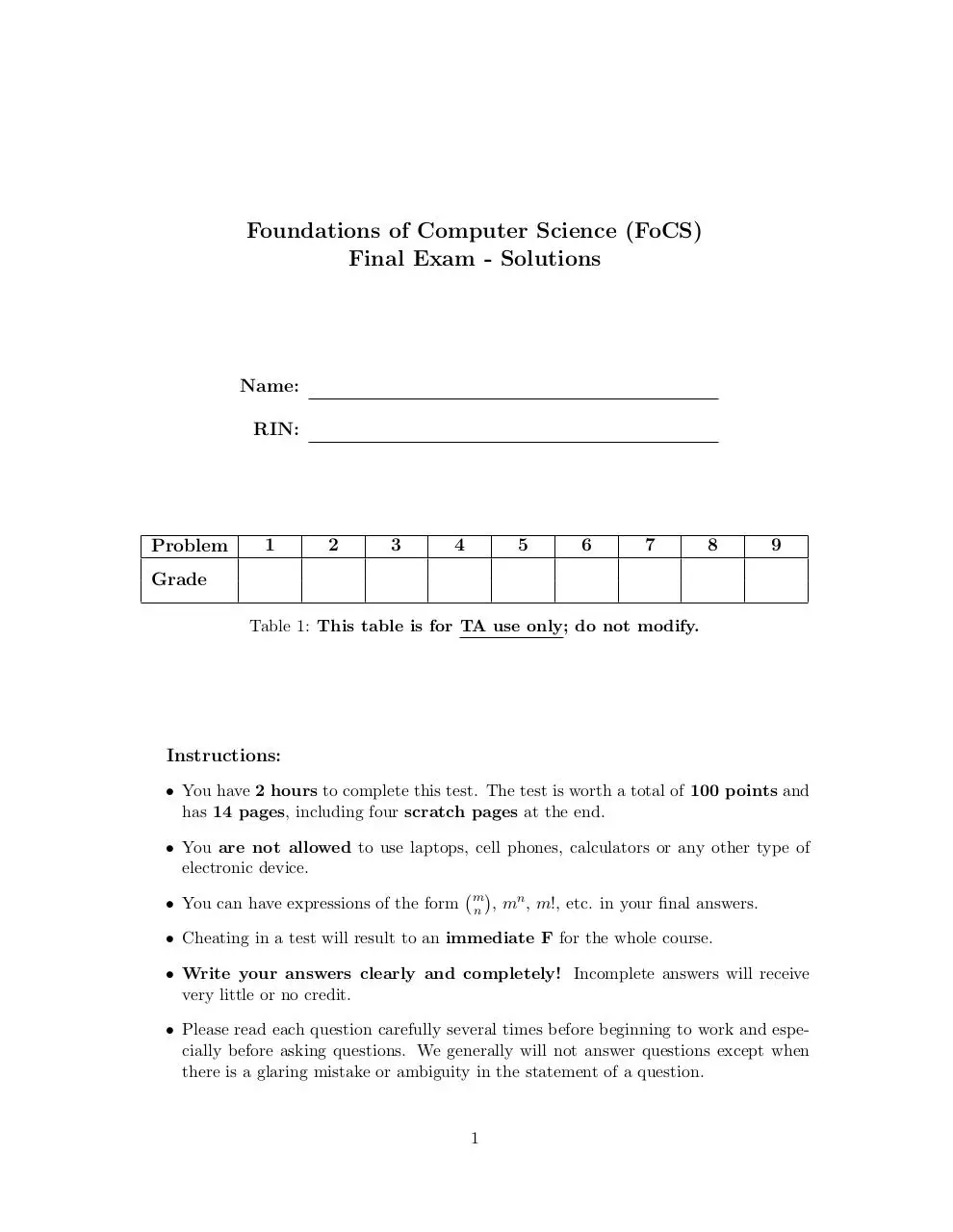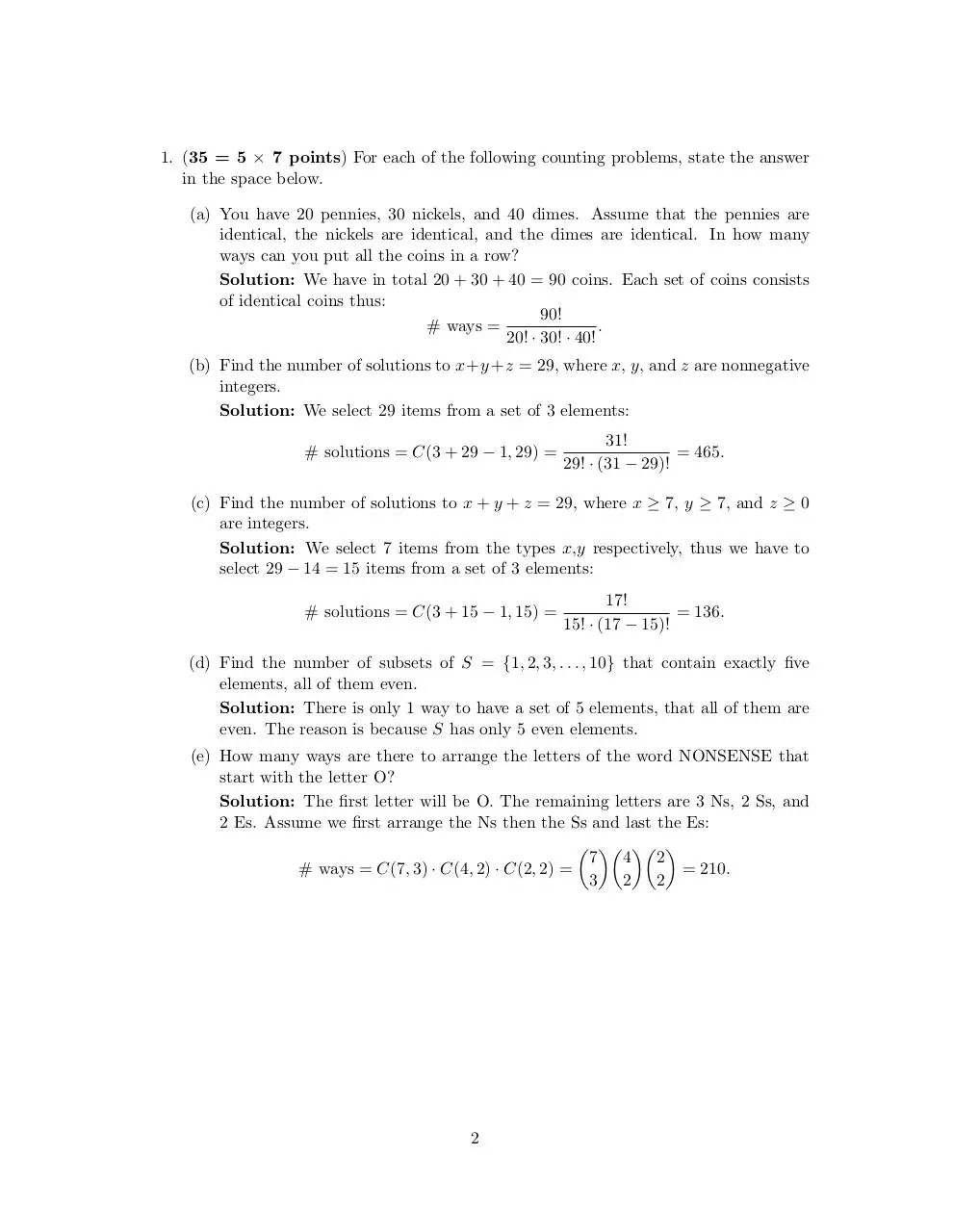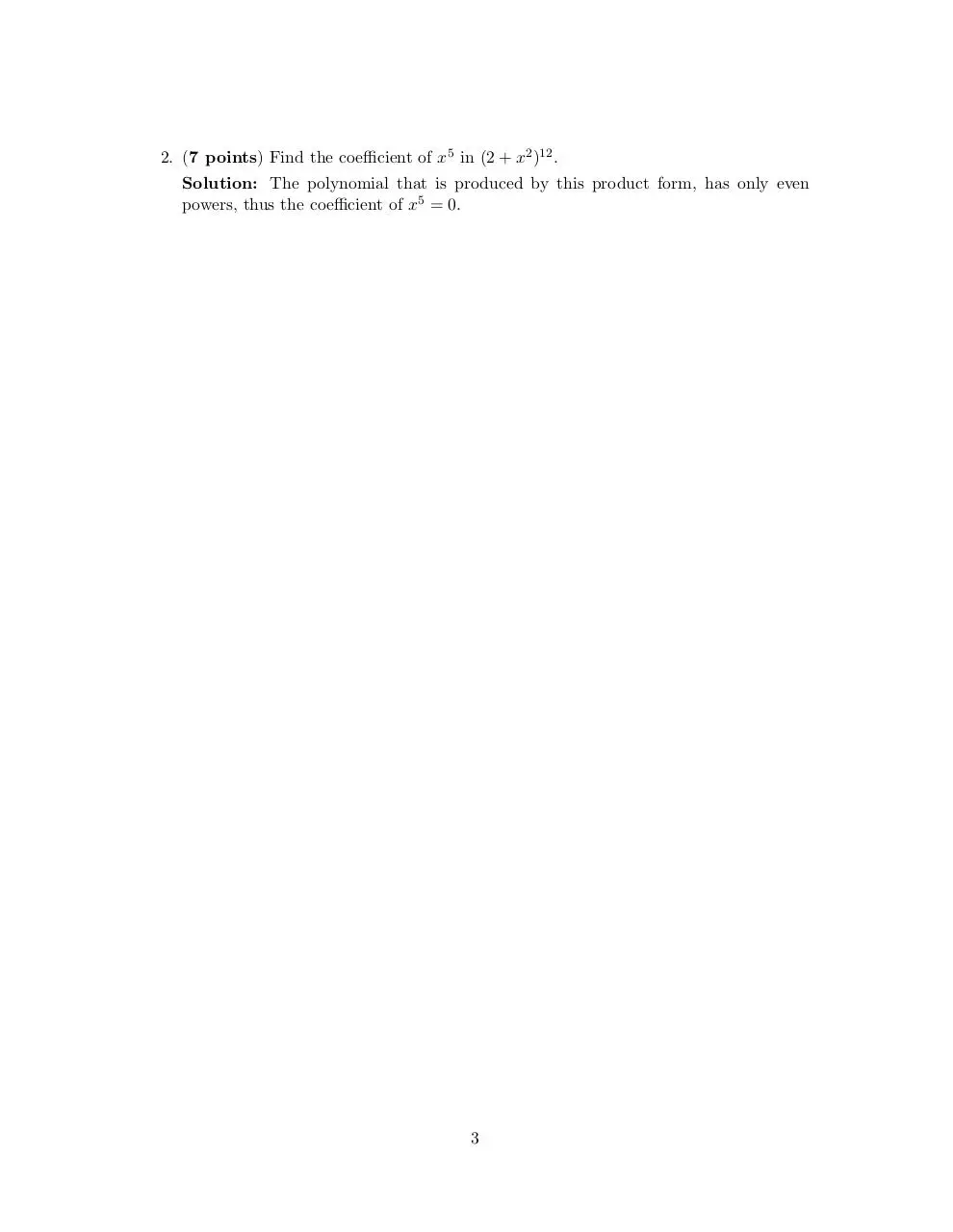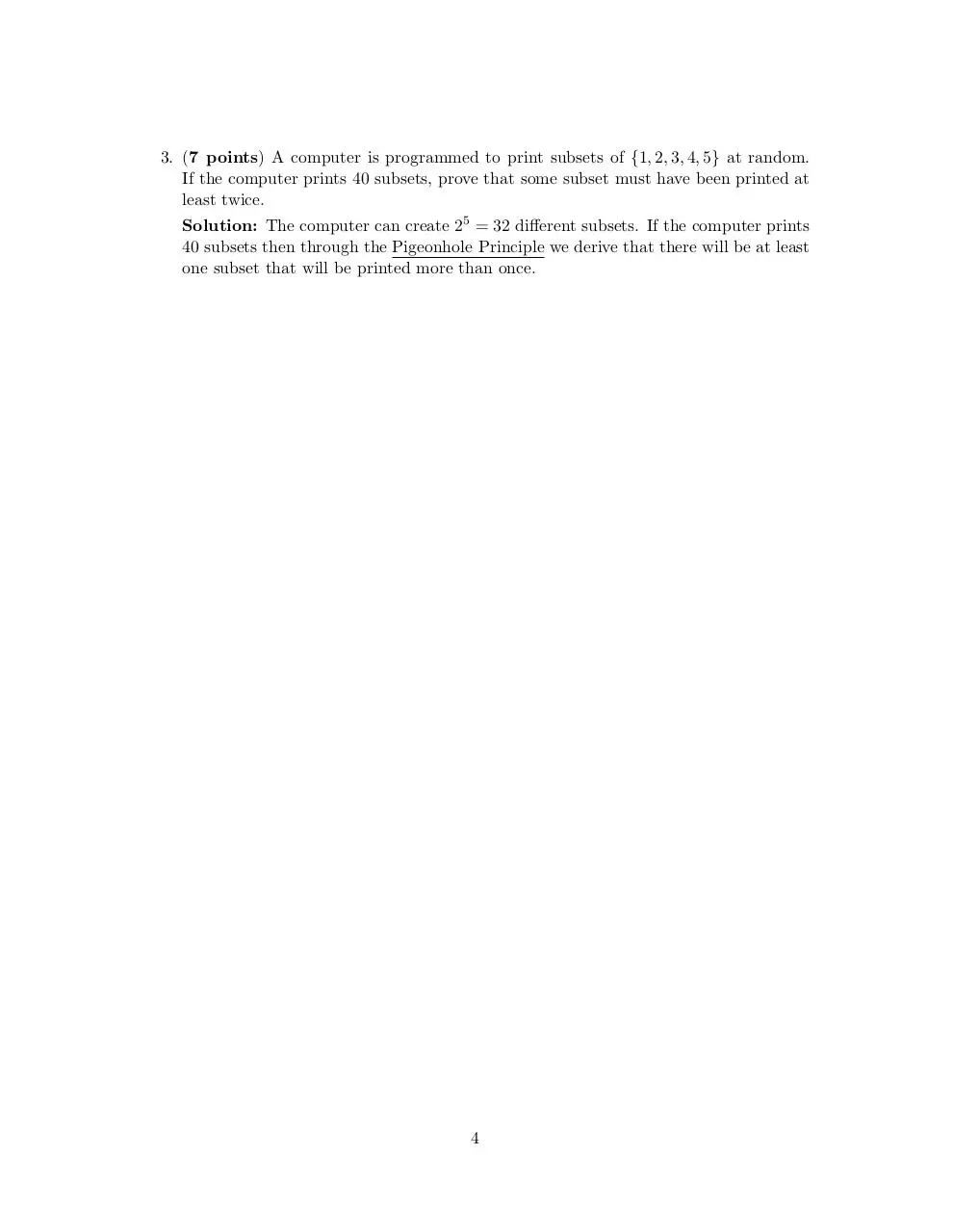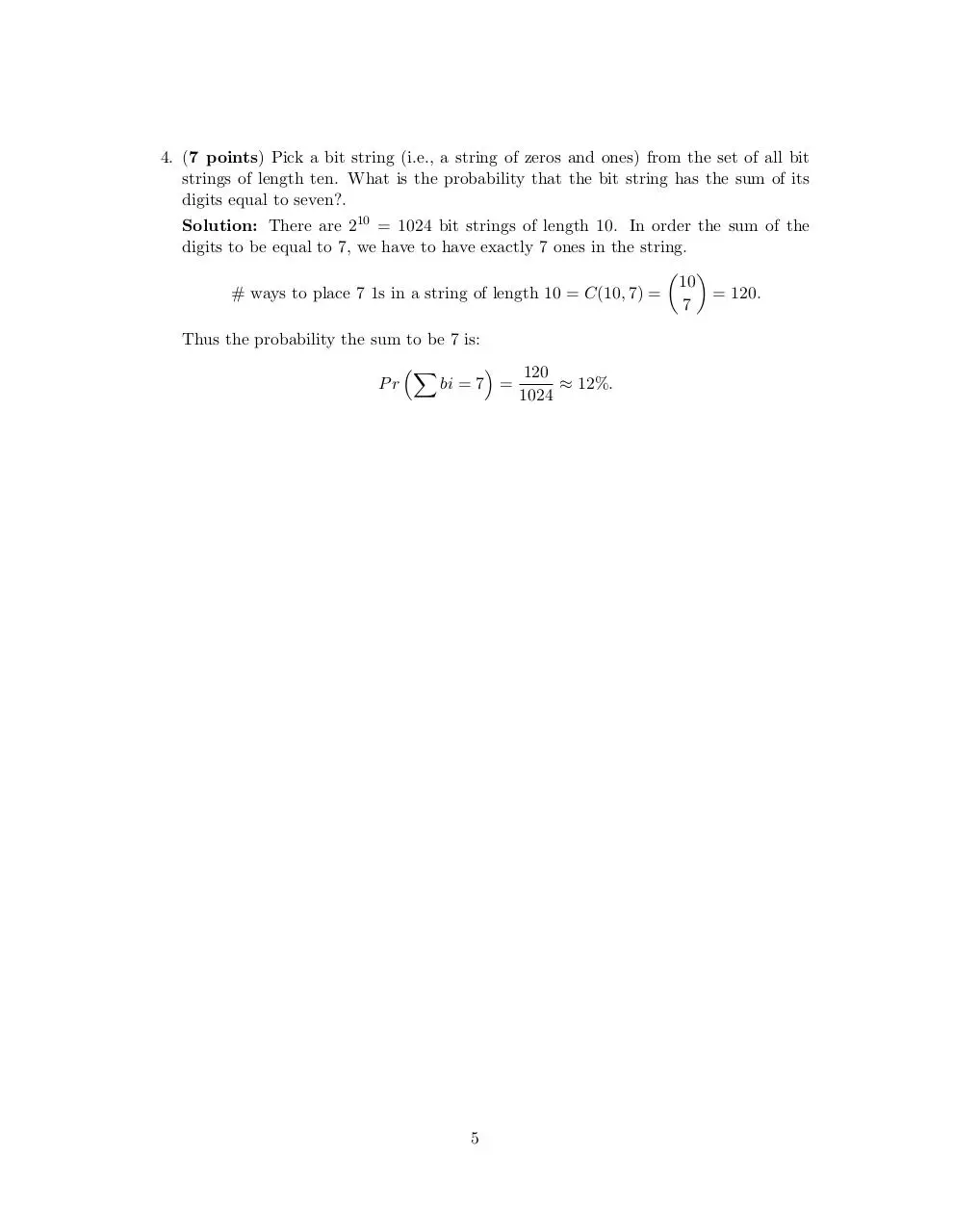### File preview

Foundations of Computer Science (FoCS)
Final Exam - Solutions

Name:
RIN:

Problem

1

2

3

4

5

6

7

8

9

Table 1: This table is for TA use only; do not modify.

Instructions:
• You have 2 hours to complete this test. The test is worth a total of 100 points and
has 14 pages, including four scratch pages at the end.
• You are not allowed to use laptops, cell phones, calculators or any other type of
electronic device.
 n
• You can have expressions of the form m
• Cheating in a test will result to an immediate F for the whole course.
very little or no credit.
• Please read each question carefully several times before beginning to work and especially before asking questions. We generally will not answer questions except when
there is a glaring mistake or ambiguity in the statement of a question.

1

1. (35 = 5 × 7 points) For each of the following counting problems, state the answer
in the space below.
(a) You have 20 pennies, 30 nickels, and 40 dimes. Assume that the pennies are
identical, the nickels are identical, and the dimes are identical. In how many
ways can you put all the coins in a row?
Solution: We have in total 20 + 30 + 40 = 90 coins. Each set of coins consists
of identical coins thus:
90!
# ways =
.
20! · 30! · 40!
(b) Find the number of solutions to x+y +z = 29, where x, y, and z are nonnegative
integers.
Solution: We select 29 items from a set of 3 elements:
# solutions = C(3 + 29 − 1, 29) =

31!
= 465.
29! · (31 − 29)!

(c) Find the number of solutions to x + y + z = 29, where x ≥ 7, y ≥ 7, and z ≥ 0
are integers.
Solution: We select 7 items from the types x,y respectively, thus we have to
select 29 − 14 = 15 items from a set of 3 elements:
# solutions = C(3 + 15 − 1, 15) =

17!
= 136.
15! · (17 − 15)!

(d) Find the number of subsets of S = {1, 2, 3, . . . , 10} that contain exactly five
elements, all of them even.
Solution: There is only 1 way to have a set of 5 elements, that all of them are
even. The reason is because S has only 5 even elements.
(e) How many ways are there to arrange the letters of the word NONSENSE that
Solution: The first letter will be O. The remaining letters are 3 Ns, 2 Ss, and
2 Es. Assume we first arrange the Ns then the Ss and last the Es:
   
7 4 2
# ways = C(7, 3) · C(4, 2) · C(2, 2) =
= 210.
3 2 2

2

2. (7 points) Find the coefficient of x5 in (2 + x2 )12 .
Solution: The polynomial that is produced by this product form, has only even
powers, thus the coefficient of x5 = 0.

3

3. (7 points) A computer is programmed to print subsets of {1, 2, 3, 4, 5} at random.
If the computer prints 40 subsets, prove that some subset must have been printed at
least twice.
Solution: The computer can create 25 = 32 different subsets. If the computer prints
40 subsets then through the Pigeonhole Principle we derive that there will be at least
one subset that will be printed more than once.

4

4. (7 points) Pick a bit string (i.e., a string of zeros and ones) from the set of all bit
strings of length ten. What is the probability that the bit string has the sum of its
digits equal to seven?.
Solution: There are 210 = 1024 bit strings of length 10. In order the sum of the
digits to be equal to 7, we have to have exactly 7 ones in the string.
 
10
# ways to place 7 1s in a string of length 10 = C(10, 7) =
= 120.
7
Thus the probability the sum to be 7 is:
Pr

X


120
bi = 7 =
≈ 12%.
1024

5

5. (15 = 5 × 3 points) For each of the following statements determine whether they
are true or false.
(a) We can design an ambiguous context-free grammar for every context-free language.
Solution: TRUE


(b) The language L = an bn , 0 ≤ n ≤ 105 is regular.
Solution: TRUE
(c) A context-free grammar for the language L is ambiguous if there exists at least
one string in L that has two leftmost derivations.
Solution: TRUE
(d) Let Σ = {0}. The set of all languages over the alphabet Σ is countably infinite.
Solution: FALSE
(e) If P = N P then the set of recursive languages is equal to the set of recursively
enumerable languages.
Solution: FALSE

6

6. (7 points) Design a deterministic or non-deterministic finite automaton for the language
L = {w ∈ Σ∗ : strings in L do not contain 001 or 111} ,
where Σ = {0, 1}.
Solution: We can create the following deterministic FA:
0,1

start

s0

λ

1

1

s1
0

0

1

s2
1

1

0
0

s4

s5

0

7

s3

7. (6 points) Consider the following context-free grammar over the alphabet Σ = {a, b}:
S → abSB| λ
B → λ
Which language does it generate?
Solution: The language generated by this context-free grammar is :
L = λ, ab, abab, ababab, . . . or L = (ab)∗ .

8

8. (6 points) Let N = {0, 1, 2, 3, . . .} be the set of natural numbers. Let S = N × N. Is
Solution: The Cartesian product consists of order pairs of the form (i, j) where i ∈ N
and j ∈ N. We are going to define the following counting procedure, that counts all
ordered pairs in N × N:
(0, 0)

(0, 1)

(0, 2)

···

(1, 0)

(1, 1)

(1, 2)

···

(2, 0)

(2, 1)

(2, 2)

···

···
Thus the set S is countably infinite.

9

final.pdf (PDF, 148.89 KB)

#### HTML Code

Copy the following HTML code to share your document on a Website or Blog